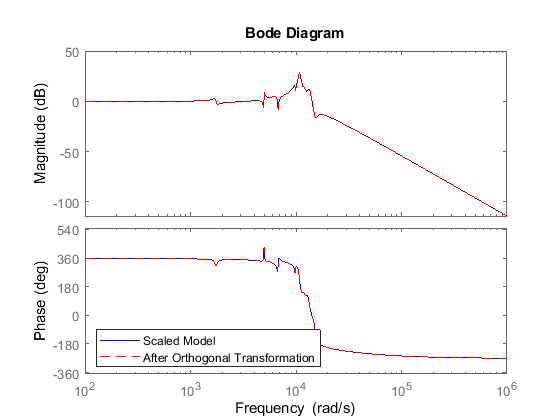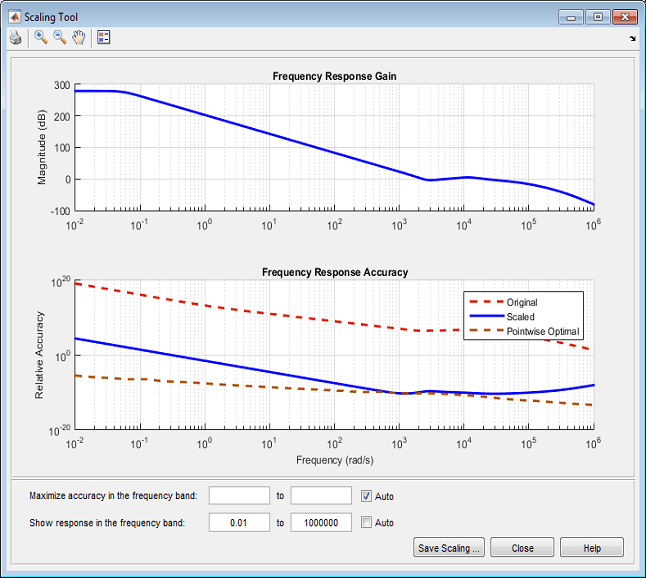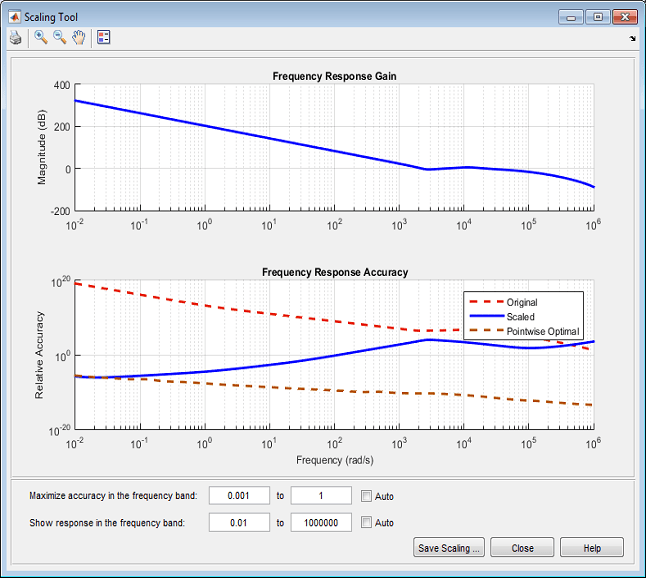# Scaling State-Space Models to Maximize Accuracy

This example shows that proper scaling of state-space models can be critical for accuracy and provides an overview of automatic and manual rescaling tools.

### Why Scaling Matters

A state-space model is well scaled when the entries of the A,B,C matrices are homogeneous in magnitude and the model characteristics are insensitive to small perturbations of A,B,C (in comparison to their norms). By contrast, a model is poorly scaled when A,B,C have both small and large entries and the model characteristics are sensitive to the small entries.

Mixing disparate time scales or unit scales can give rise to badly scaled models. Working with such models can lead to severe loss of accuracy and puzzling results. To prevent these problems, it is often necessary to rescale the state vector, that is, multiply each state by some scaling factor to reduce the numerical range and sensitivity of the model.

Poor scaling can impact the accuracy of most frequency-domain computations. State-of-the-art algorithms heavily rely on orthogonal state coordinate transformations, and such transformations introduce errors of order `eps` (the machine precision) times the norms of A,B,C. While such errors are usually negligible, they can become dominant when A,B,C are poorly scaled. To see this phenomenon, load the following example:

```load numdemo anil ```

The `anil` model is a state-space realization of a 20-th order transfer function with most of its dynamics between 1e3 and 1e5 rad/s. The coefficients of the A matrix range between 1 and 1e80 in magnitude. To simulate the effect of orthogonal transformations applied to this model, generate a random orthogonal matrix `U` and use `ss2ss` to perform the corresponding change of state coordinates:

```[U,junk] = qr(randn(20)); anil2 = ss2ss(anil,U); % perform state coordinate transformation U ```

Mathematically, `anil` and `anil2` have the same frequency response. Numerically, however, the Bode responses of `anil` and `anil2` are very different:

```bode(anil,'b',anil2,'r--') legend('Original','After Orthogonal Transformation','Location','southwest') ```
```Warning: The frequency response has poor relative accuracy. This may be because the response is nearly zero or infinite at all frequencies, or because the state-space realization is ill conditioned. Use the "prescale" command to investigate further. ```This example shows that for poorly scaled models, even orthogonal transformations can be unsafe and destroy accuracy.

### Sensitivity-Minimizing Scaling

For simple models derived from physics, you can avoid scaling issues by carefully selecting units to reduce the spread between small and large coefficients. For more complex models, however, finding a proper scaling is challenging. Ad hoc schemes such as balancing of the A matrix (see `balance`) are often useful but sometimes harmful.

The Control System Toolbox™ software provides advanced scaling algorithms that minimize the model sensitivity to small perturbations of A,B,C proportional to their norms. This helps maximize accuracy of the computed frequency response, ZPK representation, etc. The `prescale` command is the gateway to these scaling algorithms. For example, you can use `prescale` to scale the `anil` model used above:

```Scaled_anil = prescale(anil); ```

The coefficients of the A matrix now range from 1e3 to 3e7 instead of 1 to 1e80. Apply the orthogonal transformation `U` to the scaled model and compare the Bode responses:

```Scaled_anil2 = ss2ss(Scaled_anil,U); bode(Scaled_anil,'b',Scaled_anil2,'r--') legend('Scaled Model','After Orthogonal Transformation','Location','southwest') ```The Bode responses match closely now. Scaling has made orthogonal transformations safe again and you can expect good accuracy from computations involving this scaled model.

### Automatic Scaling

Most algorithms in the Control System Toolbox software automatically rescale state-space models to prevent catastrophic loss of accuracy. As a result, you are mostly insulated from scaling issues. For example, the `bode` command automatically scales incoming models so that it can safely perform orthogonal transformations to speed up the frequency response computation. Therefore, there is no need to use `prescale` before `bode` unless you want detailed information about the relative accuracy of the computed Bode response.

### Manual Scaling

While the Control System Toolbox scaling algorithm handles most models without user intervention, there are rare cases where good accuracy can't be achieved over the entire frequency range and the algorithm must trade good accuracy in one frequency band for bad accuracy in another. In such case, a warning is issued to alert you of potential inaccuracies. To illustrate this behavior, load the next example and plot its Bode response:

```load numdemo warnsys bode(warnsys,{1e-3,1e6}), grid on ```Note the warning issued by the `bode` command. This 17-th order model has dynamics near 0.01 rad/s and between 1e3 and 1e6 rad/s, separated by a 300dB gain drop. You can eliminate the warning by narrowing down the frequency range of interest, for example, to [10,1e6] rad/s:

```bode(warnsys,{10,1e6}), grid on ```This instructs the algorithm to maximizes accuracy in the interval [10,1e6]. You can also investigate the underlying accuracy tradeoff by typing:

`>> prescale(warnsys)`

This command opens the interactive Scaling Tool shown below. Set the frequency axis limits to 1e-2 to 1e6. The top plot shows the frequency response magnitude, and the bottom plot shows:

• The relative accuracy of the computed response without scaling (red)

• The relative accuracy of the computed response with scaling (blue)

• The best achievable accuracy when using independent scaling at each frequency (brown)

Any relative accuracy value greater than one signals poor accuracy.In this example, the Relative Accuracy plot shows that the scaling algorithm achieved good accuracy in the [1e3,1e6] frequency band at the expense of accuracy at low frequencies. If you only care about the frequency band [1e-3,1], you can override this default range selection and manually specify the frequency band where you want maximum accuracy. For example, enter [1e-3,1] in the edit boxes next to Maximize accuracy in the frequency band:This action updates the bottom plot and the relative accuracy of the scaled model (blue curve) is now best near 1e-2 rad/s, but is significantly worse in the [1e3,1e6] band.

### The Scaled Property of State-Space Models

The State-Space (@ss) object has a `Scaled` property to indicate when a model is already scaled. Its default value is `false`. The `prescale` command sets this property to `true`:

```sys = prescale(anil); sys.Scaled ```
```ans = logical 1 ```

Because the scaling algorithm skips models with `Scaled=true`, you can manually set the `Scaled` property to `true` when your model is well scaled and you want to eliminate the small overhead associated with scaling. If you want more control over where accuracy is maximized, you can also explicitly scale your model before using it:

```sys = prescale(warnsys,{10,1e6}); bode(sys) ```Here `warnsys` is scaled with emphasis on the frequency range [10,1e6] and `bode` does not attempt to rescale the resulting model `sys` (no more warning).

### Conclusion

Proper scaling of state-space models is important to achieve accurate results. Most Control System Toolbox commands take care of scaling automatically. You are alerted when accuracy may be compromised and you can easily correct the problem by specifying the frequency band of interest.